# Kernel congruence

(diff) ← Older revision | Latest revision (diff) | Newer revision → (diff)
of a homomorphism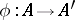of algebraic systems
The congruence (cf. Congruence (in algebra))onconsisting of all pairsfor which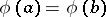. For any congruenceon an algebraic system there is a homomorphismof this system for whichis the kernel congruence. Ifis the kernel congruence of a strong homomorphismof an algebraic systemonto a system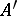, then the canonical mapping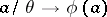, where, is an isomorphism of the quotient system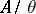onto.